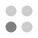• 6,774 trophies earned
• 328 Players Tracked
• 21 Total trophies
• 209 Obtainable EXP
• 1,170 Points
• 318 Platinum Club
• 318 100% Club

### Energy Cycle Edge trophies

•97.26%
•Solved first puzzle100.00%
•Puzzles 2, 3 and 4 solved99.09%
•Puzzles 5, 6 and 7 solved99.09%
•Puzzles 8, 9 and 10 solved99.09%
•Puzzles 11, 12 and 13 solved99.09%
•Puzzles 14, 15 and 16 solved98.78%
•Puzzles 17, 18 and 19 solved98.48%
•Puzzles 20, 21 and 22 solved98.48%
•Puzzles 23, 24 and 25 solved98.48%
•Puzzles 26, 27 and 28 solved97.87%
•Puzzles 29, 30 and 31 solved97.56%
•Puzzles 32, 33 and 34 solved97.56%
•Puzzles 35 and 36 solved97.56%
•Puzzles 37, 38 and 39 solved97.56%
•Puzzles 40 and 41 solved97.56%
•Puzzles 42 and 43 solved97.56%
•All of puzzles solved97.56%
•Took less than 10 seconds to solve the puzzle99.39%
•Took more than 15 minutes to solve the puzzle97.87%
•3D puzzle is solved exactly with one rotation99.09%